# Law of sines facts for kids

Kids Encyclopedia Facts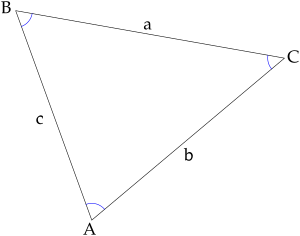A triangle labelled with the letters needed for this explanation. A, B and C are the angles. a is the side opposite A. b is the side opposite B. c is the side opposite C

The sine rule or law of sines, is a theorem in mathematics. It says that, if you have a triangle like the one in the picture, the equation below is true.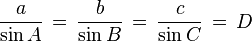$\frac{a}{\sin A} \,=\, \frac{b}{\sin B} \,=\, \frac{c}{\sin C} \,=\, D \!$

This is another version, which is also true.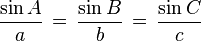$\frac{\sin A}{a} \,=\, \frac{\sin B}{b} \,=\, \frac{\sin C}{c} \!$

D is equal to the diameter of the triangle's circumcircle.

The law of sines is used to find the remaining sides of a triangle when two angles and a side are known. This is known as triangulation. However, this calculation can have a numerical error if an angle is close to 90 degrees. The law of sines can also be used when two sides and one of the angles not enclosed by the two sides are known. In some such cases, the formula gives two possible values for the enclosed angle. This is called an ambiguous case.

The law of sines is one of two trigonometric equations which is used to find lengths and angles in scalene triangles. The other is the law of cosines.

## Proof

The area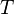$T$ of any triangle can be written as one half of its base times its height (drawn from the vertex not on the base). Depending on which side one chooses to be the base, the area can be given by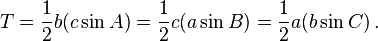$T = \frac{1}{2}b(c \sin A) = \frac{1}{2}c(a \sin B) = \frac{1}{2}a(b \sin C)\,.$

Multiplying these by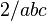$2/abc$ gives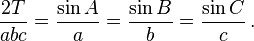$\frac{2T}{abc} = \frac{\sin A}{a} = \frac{\sin B}{b} = \frac{\sin C}{c}\,.$Law of sines Facts for Kids. Kiddle Encyclopedia.